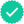Question
100%

1) For each of the data sets below, which appears to have the highest variability? Explain your answer.

a. 3, 3, 4, 4, 4, 2, 3

b. 1, 1, 1, 1, 1, 2, 2, 7

c. 4, 2, 3, 2, 3, 5, 7, 7, 7, 4, 7, 2, 7, 7, 3

2) For each of the data sets above (a,b,c), calculate the range and sample standard deviation.

3) Explain why we need to square the deviations from the mean in our calculation of the standard deviation.

Expert SolutionKnowledge Booster••